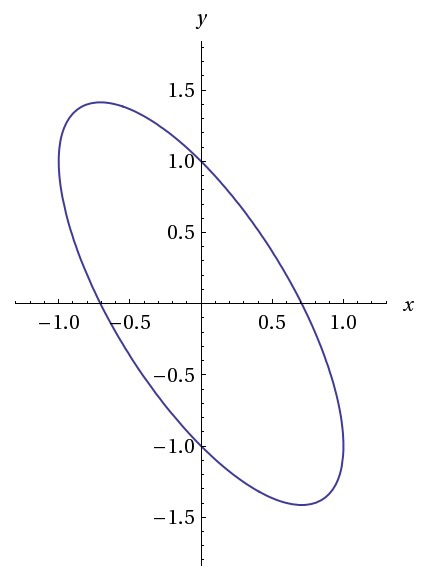Algebra

# Function Graphs: Level 4 Challenges

Given the equation $4x^2+2\sqrt{3}xy+2y^2=1$.
Through what angles $\theta \in [0,\pi]$ should the axes be rotated so that the term $xy$ is removed from the transformed equation?

Give the answer as the sum of all values of $\theta$(in degrees).The graph above shows the figure created by the function $x^2+(y+x)^2=1$.

Find the area of this tilted ellipse correct to 2 decimal places.

For each integer $n>1$, let $F(n)$ be the number of solutions to the equation $\sin{x} = \sin{(nx)}$ on the interval $[0,\pi]$. What is $\displaystyle\sum_{n=2}^{2007}$$F(n)$?

If $f(x)=\dfrac{x^2}{1-\cos x}$, where $0. Which of the following describes the function of $f(x)$?

If $f(x)$ and $g(x)$ have the same fundamental period, then so does $f(x) + g(x)$.

×